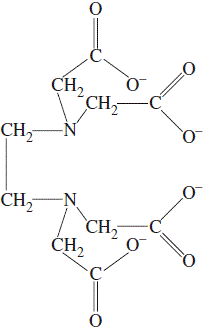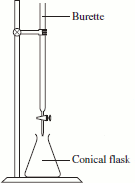Determining Total Hardness in Water by Complexometric Titration Chemistry Tutorial

Key Concepts• Calcium and magnesium salts dissolved in water cause water hardness.
• Water hardness can be measured using a titration with ethylenediaminetetraacetic acid (EDTA).
The ionised form of EDTA is shown on the right.
EDTA dissolved in water forms a colourless solution.
• At pH 10, calcium and magnesium ions form colourless, water soluble, complexes with EDTA:
calcium ion complexed by EDTA : CaEDTA2-
magnesium ion complexed by EDTA : MgEDTA2-
• An indicator, known as a metal ion indicator, is required for the titration.
Eriochromshwartz-T (Erio T) is a suitable indicator.
Eriochromshwartz-T is wine-red in hard water, but turns sky blue in the presence of free EDTA ions in the solution.
• Endpoint of the titration is when all the Ca2+ and Mg2+ ions have been complexed by the EDTA.
Before the endpoint, Ca2+ and Mg2+ are in excess, there is no free EDTA in solution.
Immediately after the endpoint, there is an excess of EDTA.
• It is essential that deionised water (water in which ions have been removed) is used in preparing all solutions, and in rinsing the conical flask.
Ca2+ and Mg2+ ions must NOT be present in the water used to prepare the solutions, or in rinsing.

No ads = no money for us = no free stuff for you!

Experimental Determination of Ca2+ and Mg2+ in Hard Water

There are 3 steps to determining the concentration of calcium and magnesium ions in hard water using the complexometric titration method with EDTA:

1. Make a standard solution of EDTA.
2. Use the standard EDTA solution to titrate the hard water.
3. Perform calculations to determine the concentration of calcium and magnesium ions in the hard water.

Preparing the Standard EDTA solution

EDTA of an analytical reagent purity is available so it can be used as a primary standard.

To prepare the EDTA standard solution:

1. Dry some EDTA at 80oC to remove any moisture.
EDTA prepared in this way will have the formula Na2H2C10H12O8N2.2H2O
Molar mass of this EDTA = (2 x 22.99) + (2 x 1.008) + (10 x 12.01) + (12 x 1.008) + (8 x 16.00) + (2 x 14.01) + 2(2 x 1.008 + 16.00)
Molar mass EDTA = 45.98 + 2.016 + 120.1 + 12.096 + 128 + 28.02 + 36.032 = 372.244 g mol-1
2. Accurately weigh out the required amount of EDTA onto a watchglass.
For example, to prepare 250 mL of a 0.010 mol L-1 solution of EDTA:

(i) Determine the moles of EDTA required:
concentration of EDTA solution = 0.010 mol L-1
volume of EDTA solution = 250.0 mL = 250.0 x 10-3 = 0.2500 L
moles EDTA = concentration (mol L-1) x volume (L) = 0.010 x 0.2500 = 2.500 x 10-3 mol

(ii) Calculate the mass of EDTA required to make 250.0 mL of 0.010 mol L-1 solution
molar mass EDTA = 372.244 g mol-1
mass EDTA required = moles x molar mass = 2.500 x 10-3 x 372.244 = 0.9306 g

3. Carefully transfer the EDTA to the volumetric flask
Place a clean, dry, glass funnel in the neck of the volumetric flask.
Use a clean, dry, spatula to transfer some of the EDTA from the watchglass to the funnel.
Use a small amount of water from a wash bottle filled with deionised water to wash the EDTA out of the funnel and into the flask.
Continue this process until no more EDTA is left on the watchglass.
Use the wash bottle to wash the spatula over the funnel to transfer any remaining EDTA into the flask.
Use the wash bottle to wash any remaining EDTA off the watchglass and into the funnel.
Use the wash bottle to give the funnel itself a final wash to ensure any remaining EDTA is washed into the flask.
4. Fill the volumetric flask to the mark with deionised water.
Deionised water can be poured into the flask using the funnel until the volume of solution in the flask is a bit below the mark on the flask.
Use a pasteur pipette to add deionised water dropwise until the bottom of the meniscus sits exactly on the mark on the flask when viewed at eye level.

Using the Standard EDTA Solution to Titrate the Hard Water1. Fill a 50.0 mL burette with the standard EDTA solution.
Rinse a clean, dry, burette with a small amount of the EDTA solution first.
Make sure the stopcock on the burette is closed!
Fill the burette with the EDTA solution to well above the 0.00 mL mark.
Place a beaker under the burette, and run the EDTA solution slowly and carefully through the burette until the bottom of the meniscus sits exactly on the 0.00 mL mark on the burette when viewed at eye level.
Check that there are no air bubbles in the solution in the burette, and that there is no "air pocket" at the tip of the burette.
Remove the beaker and dispose of its contents appropriately.
2. Rinse a 10.0 mL pipette with some of the hard water sample then pipette 10.0 mL of the hard water into a clean conical flask.
Use a clean conical flask that has been rinsed with deionised water.
3. Add 5 mL of ammonia based pH 10 buffer using a pipette1.
4. Add 2 drops of indicator such as Eriochromschwartz-T which will turn wine-red in the ammoniated water.
5. Run some EDTA through the burette and into the flask until the indicator turns sky-blue.
Keep swirling the conical flask during the titration to mix the contents.
Note the approximate endpoint of the titration.
6. Refill the burette if necessary, and repeat the titration.
Use the rough titration above as a guide as to where the endpoint is.
Run the EDTA through the burette quickly until about 10 mL before the expected endpoint.
Slowly add the EDTA 1 mL at a time until you start to see indications of a colour change in the solution, that is, a spot of blue appears before disappearing. At this point the endpoint is close.
Slowly add the EDTA dropwise until the first permanent colour change (that is, the solution goes blue and stays blue).
Record this volume of EDTA.
Use this volume of EDTA as a guide in repeating the titration.
Repeat the titration until you have 3 results (EDTA volumes) that agree to with 0.05 mL (these results are said to be concordant).

Do you know this?

Play the game now!

The Calculations

1. The balanced chemical equation for the reaction between calcium and magnesium ions and EDTA is:
Ca2+ + H2EDTA2- → CaEDTA2- + 2H+

Mg2+ + H2EDTA2- → MgEDTA2- + 2H+

So if we let M2+ be the total of Ca2+ and Mg2+ ions in solution then:

M2+ + H2EDTA2- → MEDTA2- + 2H+

Note that 1 mole of M2+ reacts with 1 mole of H2EDTA2-.
At the equivalence point of the titration:

moles M2+ = (moles Ca2+ + moles Mg2+)
= moles H2EDTA2-

2. Moles of EDTA must be calculated using the results of the titration:

Titration No.EDTA volume in burettevolume of EDTA used (mL)
starting volume (mL)final volume (mL)
Rough titration
(not used in calculations)
V(i)VfV(rough) = Vf - Vi

Titration 1V(i)VfV(1) = Vf - Vi
Titration 2V(i)VfV(2) = Vf - Vi
Titration 3V(i)VfV(3) = Vf - Vi

average titre = (V(1) + V(2) + V(3)) ÷ 3 =V(av)

moles EDTA = concentration (mol L-1) x volume (L)

concentration EDTA = concentration of standard EDTA solution = c(EDTA)
volume EDTA = average titre ÷ 1000 = V(av) x 10-3 in litres
moles EDTA = c(EDTA) x V(av) x 10-3 mol
3. Moles of calcium and magnesium ions in hard water sample (in conical flask):
From the balanced chemical equation,
moles M2+ = moles EDTA used in titration
moles M2+ = c(EDTA) x V(av) x 10-3 mol
4. Concentration of M2+ in hard water:
concentration M2+ = moles M2+ ÷ volume of water sample (L)
moles M2+ = c(EDTA) x V(av) x 10-3 mol
volume of hard water = V(w) mL = Vw x 10-3 L
concentration of M2+ = moles ÷ volume (L)
= c(EDTA) x V(av) x 10-3 ÷ V(w) x 10-3 mol L-1
Concentration of calcium and magnesium ions in the water is
= c(EDTA) x V(av) x 10-3 ÷ V(w) x 10-3 mol L-1

Do you understand this?

Take the test now!

Worked Example

A student has been given 1 L of water and asked to determine the concentration of dissolved calcium and magnesium ions in the water.
Using a 0.010 mol L-1 standard solution of EDTA, the student titrated 100.0 mL samples of the water using Eriochromshwartz-T as the indicator.
The student's titration results are shown below:

Titration No.volume EDTA (mL)
110.14
210.10
310.12

Determine the total concentration of calcium and magnesium ions in the water sample.

1. Calculate the moles of EDTA required to complex with the Ca2+ and Mg2+ ions in the water.
concentration of EDTA = 0.010 mol L-1
volume of EDTA solution = average titre = (10.14 + 10.10 + 10.12) ÷ 3 = 10.12 mL = 10.12 x 10-3 L
moles EDTA = concentration (mol L-1) x volume (L) = 0.010 x 10.12 x 10-3 = 1.012 x 10-4 mol
2. Write the equation for the reaction between EDTA and Ca2+ and Mg2+:
Ca2+ + H2EDTA2- → CaEDTA2- + 2H+
Mg2+ + H2EDTA2- → MgEDTA2- + 2H+
Let M2+ = Ca2+ + Mg2+, then
M2+ + H2EDTA2- → MEDTA2- + 2H+
3. Calculate moles of M2+ (= Ca2+ + Mg2+) present in the 100.0 mL water sample
From the balanced chemical equation: 1 mole M2+ reacts with 1 mole EDTA
So 1.012 x 10-4 moles of EDTA react with 1.012 x 10-4 moles of M2+
4. Calculate the concentration of M2+ (= Ca2+ + Mg2+) in the water
concentration (M2+) = moles M2+ ÷ volume (L)
moles M2+ = 1.012 x 10-4 mol
volume = 100.0 mL = 100.0 x 10-3 L
concentration (M2+) = 1.012 x 10-4 ÷ 100.0 x 10-3 = 1.012 x 10-3 mol L-1
5. The total concentration of dissolved calcium ions and magnesium ions in the water is 1.012 x 10-3 mol L-1

Can you apply this?

Join AUS-e-TUTE!

Do the drill now!

1. To maintain the pH at around 10, a buffer soluton is required for the titration.
Calcium and magnesium ions are kept in solution with the aid of a weak complexing reagent such as the ammonia used in this example, or tartrate is also used.